# Hamiltonian system

(diff) ← Older revision | Latest revision (diff) | Newer revision → (diff)

A system of ordinary differential equations in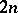unknowns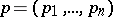( "generalized momenta" ) and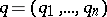( "generalized coordinates" ), of the form(1)

whereis some function of, known as the Hamilton function, or Hamiltonian, of the system (1). A Hamiltonian system is also said to be a canonical system and in the autonomous case (whenis not an explicit function of) it may be referred to as a conservative system, since in this case the function(which often has the meaning of energy) is a first integral (i.e. the energy is conserved during motion).

In mechanics, a Hamiltonian system describes a motion involving holonomic constraints and forces which have a potential (cf. Hamilton equations). Many problems in theoretical physics also lead to a Hamiltonian system or else to partial differential equations with similar properties, which may be treated as infinite-dimensional analogues of Hamiltonian systems. The equations of quantum mechanics may be put into the form of Hamiltonian systems in whichand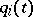are not numerical functions of time, but are rather-dependent self-adjoint linear operators which satisfy certain commutation relations. Hamiltonian systems (in the usual "finite-dimensional" sense of the word) play an important role in the study of certain asymptotic problems for partial differential equations (short-wave asymptotics for the wave equation, quasi-classical asymptotics in quantum mechanics).

Various variational principles are closely connected with Hamiltonian systems. Helmholtz' principle (see, for example, ) directly leads to a Hamiltonian system, but is used only infrequently. The most important principle is the Hamilton–Ostrogradski principle (the principle of stationary action), which directly yields the Lagrange equations (in mechanics); if certain additional conditions of non-degeneracy are met, it is possible to pass from the Lagrange equations to Hamiltonian systems using the Legendre transform (cf. Hamilton function; Hamilton equations) if the variational principle comprises first-order derivatives only. If the variational principle comprises derivatives of orders higher than one, M.V. Ostrogradski's method of passing to Hamiltonian systems becomes somewhat more complicated (see, for example, , Par. 110).

Ifis not an explicit function of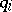, thenis a first integral. In such a case the coordinateis called cyclic (in certain cases it has the physical or the geometrical meaning of an angular variable), or ignorable. Such a coordinateand the corresponding momentum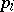may be eliminated and thus a passage is effected to a Hamiltonian system with a smaller number of unknowns. Speaking more generally, if there arefirst integrals in involution, the order of the Hamiltonian system may be reduced by(at least in a certain domain of the variables and with a transformation of the time), and hereby again a Hamiltonian system is obtained. If all coordinates are cyclic, the Hamiltonian system is said to be (completely) integrable; finding its solutions and making qualitative studies of its properties no longer involves solving differential equations.

The first integrals of a Hamiltonian system are frequently obtained from Noether's theorem: If the Lagrange function (or the Lagrangian) is invariant with respect to some continuous group of transformations, then the corresponding Hamiltonian system has first integrals of a certain type . Another general concept which sometimes makes it possible to integrate a Hamiltonian system involves passing to an auxiliary partial differential equation — the so-called Hamilton–Jacobi equation (cf. Hamilton–Jacobi theory). By separation of variables in suitable coordinates it is sometimes possible to find a complete integral of the latter equation, and according to Jacobi's theorem the Hamiltonian system is then relatively easily integrated. (The connection between the Hamiltonian system and the Hamilton–Jacobi equation is reversible: Solving the Hamilton–Jacobi equation is reduced to the integration of the corresponding Hamiltonian system. Historically, this relation, as well as the analogy between mechanics and geometrical optics , , , favoured the discovery of Hamiltonian systems (the Huygens principle in optics immediately yields the eikonal equation, which is an equation of Hamilton–Jacobi type). Recently one more method for integrating has been developed: the-pair (cf. , ). (Although this method is not necessarily related to Hamiltonian systems, its main applications are precisely to Hamiltonian systems.)

Specific methods of approximate integration and qualitative study of the properties of solutions have been developed for Hamiltonian systems which are close to integrable. Similar methods are also used to study the behaviour of trajectories of Hamiltonian systems in a neighbourhood of an equilibrium position, and of periodic or quasi-periodic solutions. Methods not specifically connected with Hamiltonian systems may also be employed, but even then the specific nature of Hamiltonian systems may well simplify the computations or, on the contrary, complicate the problem (since, from the point of view of a general method, the Hamiltonian system may prove "exceptional" (as is the case, for example, with (structural) stability). See Adiabatic invariant; Small parameter, method of the; Small denominators; Averaging).

Let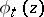be the solution of the Hamiltonian system (1) that passes through the point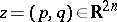at the time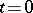. The Hamiltonian system is unambiguously characterized by the fact that the (local) diffeomorphisms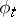transform the exterior differential form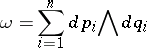(2)

(the so-called Poincaré integral invariant) to; this fact was noted, albeit in different terms, by Ostrogradski and H. Helmholtz; the latter spoke of "the property of mutuality" . Diffeomorphisms which preserveare called canonical transformations or canonical changes of variables; not only do they naturally arise from Hamiltonian systems, but they convert any Hamiltonian system to a Hamiltonian system. (This property is also shared by generalized canonical transformations, the transition to which is not a very essential extension of the class of transformations under consideration.) The Hamiltonian of the new system is readily expressed in terms of the old Hamiltonian and the generating functionof the canonical transformation (the latter is conveniently defined by means of this). Thus, if a Hamiltonian system is given, it is possible, by effecting a canonical change of variables, to conduct computations involving the two functionsandonly (whereas in the general case a change of variables in a system of differential equations would involve a much larger number of functions); this is what constitutes the so-called canonical formalism, which considerably simplifies computations carried out within its range of applicability. It is widely used in celestial mechanics and in theoretical physics.

The definition of a Hamiltonian system in terms of an integral invariant leads to a natural extension, when the form (2) in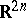is replaced by an exterior differential formof degree 2, defined on some manifoldof even dimension; here,is closed (the exterior differential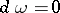) and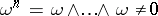everywhere (one then says that a symplectic structure is defined on). Even more general is the treatment of a Hamiltonian system not as a dynamical system, but as a congruence, where the trajectory is considered simply as a family of lines with definite properties while disregarding the speed of motion along them. (System (1) may be included in this scheme by considering the graphs of the solutions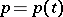,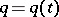in the space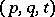; here, the important object is the Poincaré–Cartan integral invariant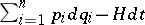rather than (2).) Strictly speaking, if the order of the Hamiltonian system (1) is reduced with the aid of first integrals, the resulting Hamiltonian system has this generalized meaning .

Little is known (except for the problems of the local behaviour of trajectories, discussed above) about the properties of Hamiltonian systems that are not integrable or not close to integrable. The preservation of the volume element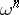follows from the preservation of(the Liouville theorem; the converse is not true, except in low dimensions). Accordingly, Hamiltonian systems are systems with an invariant measure; such systems are studied in ergodic theory.

For recent surveys see .

How to Cite This Entry:
Hamiltonian system. Encyclopedia of Mathematics. URL: http://encyclopediaofmath.org/index.php?title=Hamiltonian_system&oldid=13766
This article was adapted from an original article by D.V. Anosov (originator), which appeared in Encyclopedia of Mathematics - ISBN 1402006098. See original article